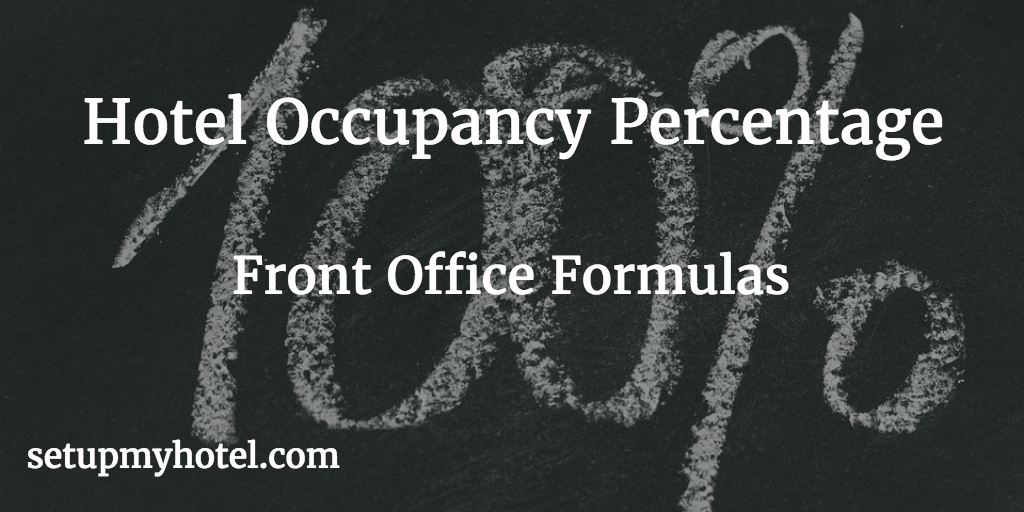# FO Formula - Hotel Occupancy Percentage | Occupancy Ratio Calculation

Occupancy Percentage is the most commonly used operating ratio in the hotel front office, The Occupancy percentage indicates the proportion of rooms either sold or occupied to the number of rooms available for the selected date or period.

The Formula for Occupancy Percentage:

Occupancy Percentage= (Number of Rooms Occupied) / (Total Number of Rooms Available for sale) * 100

There are generally two ways used to calculate the occupancy percentage in hotel, one by the number of Rooms Occupied and another by the Rooms Sold (Rooms Sold = Occupied rooms - Complimentary and House use)

Hotel Occupancy Percentage Calculator

Total Rooms Available:

Total Rooms Occupied:

Total Comp & House:

Occupancy % (Occupied Rooms)=

Occupancy % (Sold Rooms)=

Also at some hotel Available Rooms are taken after reducing the OOO (Out of Order Rooms) ie Sellable Rooms = Available Rooms - Out Of Order Rooms. Other hotels always take all rooms in their hotel as Available Rooms because this gives a consistent base on which the occupancy is measured.

1) Example Occupancy Percentage Calculation (Based on Rooms Occupied)

Total Number of Rooms Available in the hotel             = 215

Number of Rooms Occupied on 10th September 2017 = 207

Hotel's Occupancy Percentage = 207 / 215 *100

= 96.28 %

2) Example Occupancy Percentage Calculation (Based on Rooms Sold)

Total Number of Rooms Available in the hotel             = 215

Number of Rooms Occupied on 10th September 2017 = 207

Total House Use and Complimentary Rooms = 2

Hotel's Occupancy Percentage = (207 - 2) / 215  *100

= 95.34 %

3) Example Occupancy Percentage Calculation (Based on Available Rooms)

Total Number of Rooms in the hotel                          = 215

Number of Rooms Occupied on 10th September 2017 = 207

Hotel's Occupancy Percentage = 207 / 215 *100

= 96.28 %

4) Example Occupancy Percentage Calculation (Based on Sellable Rooms)

Total Number of Rooms Available in the hotel             = 215

Number of Rooms Occupied on 10th September 2017 = 207

Total Out of Order (OOO) Rooms = 5

Hotel's Occupancy Percentage = 207 / (215 - 5)  *100

= 98.57 %